Home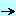papersoop_componentsdelphi_virtual_constructor

## Delphi Virtual Constructor - Felix John COLIBRI.

• abstract : VIRTUAL CONSTRUCTORS together with CLASS references and dynamic Packages allow the separation between a main project and modules compiled and linked in later.
• key words : VIRTUAL CONSTRUCTORS - CLASS references - dynamic Packages - LoadLibrary - RegisterClass - object oriented programming
• software used : Windows XP, Delphi 6
• hardware used : Pentium 2.800Mhz, 512 M memory, 140 G hard disc
• scope : Delphi 5, Delphi 6, Delphi 7, Delphi 2005, Delphi 2006, Turbo Delphi, Delphi 2007
• level : Delphi developer
• plan :

## 1 - Why Virtual Constructors ?

The Turbo Pascal 5.5 rule was "Constructors are NEVER virtual, since each constructor has to create the instance from a single class". Technically, the pointer at the beginning of the object was used to nest a reference to the Virtual Method Table, and this reference had to be specific to this class. Just the opposite of VIRTUAL and polymorphism.

When Delphi came along, Virtual Constructors were possible. We were too busy at the time with so many other things to understand and master, that for a long period all my CLASS's CONSTRUCTOR were PUBLIC VIRTUAL, which did not seem to hurt.

Later some papers told us that this VIRTUAL CONSTRUCTOR business was at the heart of the IDE machinery, allowing the developer to add new components without having to recompile the IDE.

I only understood the working of this concept when we reengineered our old DOS drawing tool to upgrade it to a full fledged Palette / Inspector / Design surface vector graphic and UML editor.

The objective of this paper is to present a minimal example of how Delphi is able to instantiate new components without having to recompile the IDE. And of course, the whole thing is linked to VIRTUAL CONSTRUCTORs !

## 2 - Virtual Constructor, Class Reference, Package

### 2.1 - The Example

We will build a tiny editor, where the user can click a figure icon (Ellipse, Rectangle, Triangle) and then draw the corresponding shape on a tPaintBox

We will present two versions:

• the first solution will simply gather all the required UNITs and CLASSes, every pieces being linked together by USES clauses.
• the second solution will present an application containing the ancestor CLASSes. And the different figures will be appended later, without touching (recompiling) the drawing application.

### 2.2 - The Tiny Shape Editor

We first create the three shape CLASSes. In order to be able to add them to some structure (list, tree), we derive the shape from the same abstract base shape, defined by:

 c_base_figure= class(c_basic_object)                  protected                    m_x, m_y, m_width, m_height: Integer;                  public                    Constructor create_figure(p_name: String;                        p_x, p_y, p_width, p_height: Integer); Virtual;                    procedure draw_figure(p_c_canvas: tCanvas); Virtual; Abstract;                 end; // c_base_figure

And here is the definition of one of the descendent shape:

 c_ellipse_figure= class(c_base_figure)                     public                       Constructor create_figure(p_name: String;                           p_x, p_y, p_width, p_height: Integer); Override;                       procedure draw_figure(p_c_canvas: tCanvas); Override;                    end; // c_ellipse_figure

The main program contains:

• a drawing surface (a tPaintBox)
• 3 tSpeedButtons allowing to select one of the three possible shapes
• the MouseDown and MouseUp events allow to draw the chosen shapes

The simplest implementation would simply remember which tSpeedButton was clicked, and the corresponding shape would be created and drawn. For instance:

 var g_figure_name: String; procedure TForm1.ellipse_speedbutton_Click(Sender: TObject);   begin     g_figure_name= 'ellipse';   end; // ellipse_speedbutton_Click var g_start_x, g_start_y: Integer; procedure TForm1.PaintBox1MouseDown(Sender: TObject; Button: TMouseButton;     Shift: TShiftState; X, Y: Integer);   begin     g_start_x:= X;     g_start_y:= Y;   end; // PaintBox1MouseDown procedure TForm1.PaintBox1MouseUp(Sender: TObject; Button: TMouseButton;     Shift: TShiftState; X, Y: Integer);   var l_c_current_figure: c_base_figure;   begin     if g_figure_name= 'ellipse'       then l_c_current_figure:= g_c_base_figure_ref.create_figure('ellipse',           g_start_x, g_start_y, X+ 1- g_start_x, Y+ 1- g_start_y);     l_c_current_figure.draw_figure(PaintBox1.Canvas);     l_c_current_figure.Free;   end; // PaintBox1MouseUp

### 2.3 - Using CLASS reference

In order to create one of the shape, we used a simple string comparison. An enumerated value would have been another possibility.

We can also use a CLASS reference. In the base figure unit, we declare the c_base_figure_ref as a CLASS OF type:

 unit u_c_base_figure;   interface     uses Graphics, u_c_basic_object;     type c_base_figure= class(c_basic_object)                           protected                             m_x, m_y, m_width, m_height: Integer;                           public                             Constructor create_figure(p_name: String;                                 p_x, p_y, p_width, p_height: Integer); Virtual;                             procedure draw_figure(p_c_canvas: tCanvas);                                  Virtual; Abstract;                          end; // c_base_figure         c_base_figure_ref= Class of c_base_figure;

And in the main program:

• we declare a variable of type c_base_figure_ref

 var g_c_base_figure_ref: c_base_figure_ref= Nil;

This variable can be assigned any CLASS TYPE in the c_base_figure hierarchy: c_base_figure, or c_ellipse_figure, c_rectangle_figure, c_triangle_figure, or any of a later descendent.

• for each tSpeedButton click, we assign to this variable the shape type

 procedure TForm1.ellipse_speedbutton_Click(Sender: TObject);   begin     g_c_base_figure_ref:= c_ellipse_figure;   end; // ellipse_speedbutton_Click

• we use this type to create the corresponding shape

 procedure TForm1.PaintBox1MouseUp(Sender: TObject; Button: TMouseButton;     Shift: TShiftState; X, Y: Integer);   var l_c_current_figure: c_base_figure;   begin     l_c_current_figure:= g_c_base_figure_ref.create_figure('fig',         g_start_x, g_start_y, X+ 1- g_start_x, Y+ 1- g_start_y);     l_c_current_figure.draw_figure(PaintBox1.Canvas);     l_c_current_figure.Free;   end; // PaintBox1MouseUp

In fact, the g_c_base_figure_ref simply replaced the CLASS TYPE, which was c_ellipse_figure in our first trial

Here is a snapshot of the project: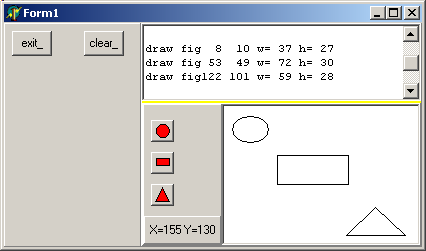However we have not yet achieved complete separation between the main program and all the U_C_xxx_FIGURE units, since they have all been imported in the USES clause of the main tForm.

### 2.4 - Using dynamic Packages

#### 2.4.1 - The basic concept

The complete separation can be achieved using Packages, because Packages can be loaded using their file name, using the LoadPackage routine.

So

• we create a Package which encapsulates our shape UNITs (separately or several in one Package)
• we place on disk a .TXT file containing the list of all package names
• the main project reads the Package list, and
• for each shape, creates a SpeedButton with a link to the c_xxx_figure shape CLASS

#### 2.4.2 - The c_class_list

The simplest would be to place the CLASS TYPE in each tSpeedButton Tag property. But this is too closely related to our graphic example. The classic solution is to build a <class_name, class_type> list, using any Delphi container CLASS (tStringList in our case).

Here is the definition of our c_class_list, using the usual tStringList encapsulation technique:

 c_class= Class(c_basic_object)            // -- m_name: the class name            m_c_base_figure_ref: c_base_figure_ref;            Constructor create_class(p_name: String;                p_c_base_figure_ref: c_base_figure_ref);            function f_display_class: String;            function f_c_self: c_class;          end; // c_class c_class_list=Class(c_basic_object)                m_c_class_list: tStringList;                Constructor create_class_list(p_name: String);                function f_class_count: Integer;                function f_c_class(p_class_index: Integer): c_class;                function f_index_of(p_class_name: String): Integer;                function f_c_find_by_class(p_class_name: String): c_class;                procedure add_class(p_class_name: String; p_c_class: c_class);                function f_c_add_class(p_class_name: String;                      p_c_base_figure_ref: c_base_figure_ref): c_class;                procedure display_class_list;                procedure load_packages_register_classes(p_full_file_name: String);                function f_c_base_figure_ref(p_class_name: String): c_base_figure_ref;                Destructor Destroy; Override;              end; // c_class_list

Two methods are of interest here:

• the function which returns the c_base_figure_ref:

 function c_class_list.f_c_base_figure_ref(p_class_name: String): c_base_figure_ref;   var l_c_class: c_class;   begin     l_c_class:= f_c_find_by_class(p_class_name);     Result:= l_c_class.m_c_base_figure_ref;   end; // f_c_base_figure_ref

When we ask for the 'c_ellipse_figure', the function returns the reference to the c_ellipse_figure CLASS, which in fact converts a string into a CLASS reference

• the initialization of the list from a .TXT file:

 procedure c_class_list.load_packages_register_classes(p_full_file_name: String);   type t_pr_register_figure= Procedure(p_c_class_list: c_class_list);   var l_package_file_name: String;       l_class_index: Integer;       l_package_handle: tHandle;       l_pr_register_figure: t_pr_register_figure;   begin     with tStringList.Create do     begin       LoadFromFile(p_full_file_name);       for l_class_index:= 0 to Count- 1 do       begin         l_package_file_name:= 'pk_'+ Strings[l_class_index]+ '_figure.bpl';         l_package_handle:= LoadPackage(l_package_file_name);         l_pr_register_figure:=             GetProcAddress(l_package_handle, 'register_figure');         // -- this will add the  item to the c_class_list         l_pr_register_figure(Self);       end; // for l_class_index       Free;     end; // with tStringList   end; // load_packages_register_classes

This works like this:

• on disk, we save a file with the shape names:

 ellipse     rectangle     triangle

• this file is loaded by our method, and for each xxx shape name
• a package PK_xxx_FIGURE.BPL is loaded
• the address of the register_figure procedure is computed
• this procedure is called
In each package, the register_figure adds the package's figure CLASSes to the c_class_list. Here is the register_figure in the PK_ELLIPSE_FIGURE package:

 procedure register_figure(p_c_class_list: c_class_list);   begin     p_c_class_list.f_c_add_class('c_ellipse_figure', c_ellipse_figure);   end; // register_figure

#### 2.4.3 - The figure Packages

Here is our pk_ellipse_figure Package:

 package pk_ellipse_figure; {\$R *.res} {\$ALIGN 8} // ...ooo... requires   rtl,   vcl,   vclx; contains   u_c_base_figure in '..\..\units\u_c_base_figure.pas',   u_c_ellipse_figure in '..\..\units\u_c_ellipse_figure.pas'; end.

And the figure UNITs are those of our first trial, but with the register_figure procedure:

 unit u_c_ellipse_figure;   interface     uses Graphics, u_c_base_figure         , u_c_class_list         ;     type c_ellipse_figure= class(c_base_figure)                             public                               Constructor create_figure(p_name: String;                                   p_x, p_y, p_width, p_height: Integer); Override;                               procedure draw_figure(p_c_canvas: tCanvas); Override;                            end; // c_ellipse_figure     procedure register_figure(p_c_class_list: c_class_list);     Exports register_figure;   implementation     // -- ...ooo...

#### 2.4.4 - The main tForm

In the main tForm
• we use a tButton to create the tSpeedButton and build the c_class_list
• each clic will provide a figure name, which will be used to create the shape
 unit u_dynamic_loading;   interface   uses // ...ooo...   type TForm1= class(TForm)                    // --- ...ooo...                  private                    procedure figure_speedbutton_Click(Sender: TObject);                end; // TForm1   implementation     uses u_c_base_figure, u_c_class_list;     {\$R *.DFM}     var g_c_class_list: c_class_list= Nil;     // -- register     procedure TForm1.load_packages_Click(Sender: TObject);       procedure create_speed_buttons;         var l_speed_top, l_speed_left: Integer;             l_class_index: Integer;             l_raw_name, l_class_name: String;             l_c_bitmap: tBitMap;         begin           l_speed_left:= 5; l_speed_top:= 5;           with tStringList.Create do           begin             LoadFromFile('class_list.txt');             for l_class_index:= 0 to Count- 1 do               with tSpeedButton.Create(Self) do               begin                 l_raw_name:= Strings[l_class_index];                 l_class_name:= 'c_'+ l_raw_name+ '_figure';                 Name:= l_class_name;                 Parent:= speed_button_panel_;                 Left:= l_speed_left;                 Top:= l_speed_top;                 l_c_bitmap:= tBitmap.Create;                 l_c_bitmap.LoadFromFile('glyph\'+ l_raw_name+ '.BMP');                 Glyph:= l_c_bitmap;                 OnClick:= figure_speedbutton_Click;                 Inc(l_speed_top, Height+ 5);               end; // for l_class_index, create             Free;           end; // with tStringList         end; // create_speed_buttons       begin // load_packages_Click         create_speed_buttons;         g_c_class_list:= c_class_list.create_class_list('class_list');         g_c_class_list.load_packages_register_classes('class_list.txt');       end; // load_packages_Click     // -- add figures     var g_figure_name: String= '';     procedure TForm1.figure_speedbutton_Click(Sender: TObject);       begin         g_figure_name:= (Sender as tSpeedButton).Name;       end; // figure_speedbutton_Click     var g_start_x, g_start_y: Integer;     procedure TForm1.PaintBox1MouseDown(Sender: TObject; Button: TMouseButton;         Shift: TShiftState; X, Y: Integer);       begin         g_start_x:= X;         g_start_y:= Y;       end; // ScrollBox1MouseDown     procedure TForm1.PaintBox1MouseUp(Sender: TObject; Button: TMouseButton;         Shift: TShiftState; X, Y: Integer);       var l_c_figure_ref: c_base_figure_ref;           l_c_current_figure: c_base_figure;       begin         l_c_figure_ref:= g_c_class_list.f_c_base_figure_ref(g_figure_name);         if l_c_figure_ref= Nil           then display_bug_halt('figure_ref_nil');         l_c_current_figure:= l_c_figure_ref.create_figure(g_figure_name,             g_start_x, g_start_y, X+ 1- g_start_x, Y+ 1- g_start_y);         l_c_current_figure.draw_figure(PaintBox1.Canvas);         l_c_current_figure.Free;       end; // ScrollBox1MouseUp     end.

And:

• the tSpeedButton are created using the CLASS_LIST.TXT file. Each tSpeedButton
• has a Name which is the 'c_xxx_figure' string
• the glyph is loaded from an XXX.BMP image
• the OnClick event is tied to a generic figure_speedbutton_click event which returns the Name of the Sender parameter
• the 'c_xxx_figure' string is used to locate the c_xxx_figure CLASS reference, which will create the corresponding shape

Here is a snapshot of the project after compilation: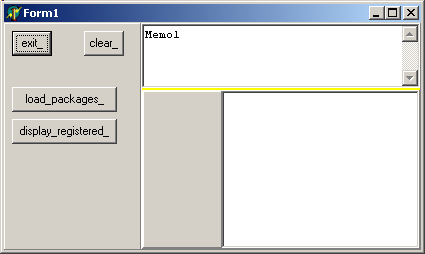and the same after we clicked "load_packages"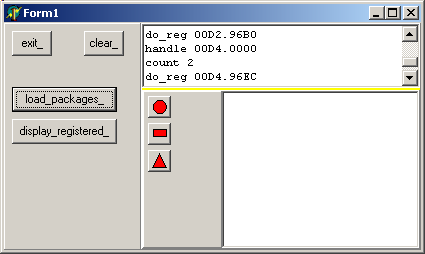#### 2.4.5 - The Overall Picture

We have separated our application in two parts:
• a main tForm which only imports an ancestor c_base_figure CLASS, and a c_class_list. This tForm loads a .TXT file which can be written after the compilation
• several Packages, which can be compiled much later, even without the sources of the main project.

We can display this on the following UML Class Diagram - like graphic: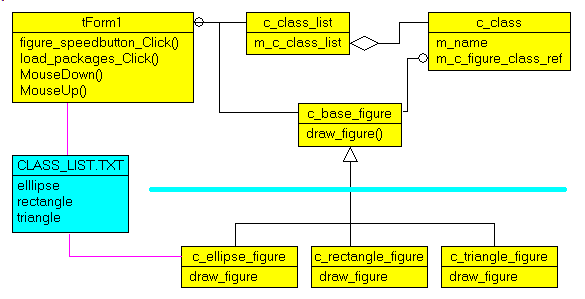To drive the point home

• the main project can create any c_base_figure descendent because it can instantiate such a descendent using a CLASS reference
• the CLASS reference is searched in a <string, class_ref> list which can be build at runtime, using information updated after the main project compilation
• we can add any kind of c_base_figure descendent which contains:
• the VIRTUAL methods of the ancestor
• a register_figure method which will be used to initialize the <string, class_ref> link
• a .TXT file is used as the link between the main project and all the descendent shapes

The whole thing works because:
• once we hold a CLASS reference

 my_c_figure_ref:= c_ellipse_figure;

• we can create the descendent CLASS using a VIRTUAL constructor:

 my_c_figure:= my_c_figure_ref.create_figure(g_figure_name,             g_start_x, g_start_y, X+ 1- g_start_x, Y+ 1- g_start_y);

## 3 - Application Frameworks and Plugins

Using this basic separation technique, we continued the graphic editor to get the full fledged "vector graphic / UML editor" by adding:
• the resizing plots
• the mouse movements
• an Object Inspector
• a figure container (a tree in our case)
• a streaming mechanism
The gorgeous UML diagram above was generated using this tool. And we added all kinds of utilities, like Database generation, Delphi reverse engineering and generation, but that's another story ...

To establish the link between the CLASS name and a CLASS reference, Delphi offers the RegisterClass procedure. To qualify for this procedure, a CLASS must descend from tComponent, which is not the case in our example. But there is no reason why this could not be changed. But using more primitive CLASSes and a homegrown c_class_list registering mechanism was more instructive.

Finally, the separation of a main project and several UNITs compiled later but used by the main project without any recompilation is at the heart of many useful techniques:

• the split of huge .EXE into separated modules, which can shorten link time, and also make deployment much easier (you only ship the new .BPLs)
• the split of an application into conceptual modules which can be developed by different teams. This can be called an "Application Framework", where the main project simply loads the different Packages

Here are the source code files:
• virtual_constructor.zip : the first trial with the project and the shape units (12 K)
• dynamic_loading.zip : the project and the separated packages and shape units (15 K)
To use this .ZIP
• compile the .DPR
• compile the three .DPK (making sure the .BPL will be found by the .EXE)
• execute the .EXE

The .ZIP file(s) contain:

• the main program (.DPR, .DOF, .RES), the main form (.PAS, .DFM), and any other auxiliary form
• any .TXT for parameters, samples, test data
• all units (.PAS) for units
Those .ZIP
• are self-contained: you will not need any other product (unless expressly mentioned).
• for Delphi 6 projects, can be used from any folder (the pathes are RELATIVE)
• will not modify your PC in any way beyond the path where you placed the .ZIP (no registry changes, no path creation etc).
To use the .ZIP:
• create or select any folder of your choice
• using Delphi, compile and execute
To remove the .ZIP simply delete the folder.

The Pascal code uses the Alsacian notation, which prefixes identifier by program area: K_onstant, T_ype, G_lobal, L_ocal, P_arametre, F_unction, C_lasse etc. This notation is presented in the Alsacian Notation paper.

As usual:

• we welcome any comment, criticism, enhancement, other sources or reference suggestion. Just send an e-mail to fcolibri@felix-colibri.com.
• or more simply, enter your (anonymous or with your e-mail if you want an answer) comments below and clic the "send" button
 Name : E-mail : Comments * :

## 5 - The author

Felix John COLIBRI works at the Pascal Institute. Starting with Pascal in 1979, he then became involved with Object Oriented Programming, Delphi, Sql, Tcp/Ip, Html, UML. Currently, he is mainly active in the area of custom software development (new projects, maintenance, audits, BDE migration, Delphi Xe_n migrations, refactoring), Delphi Consulting and Delph training. His web site features tutorials, technical papers about programming with full downloadable source code, and the description and calendar of forthcoming Delphi, FireBird, Tcp/IP, Web Services, OOP  /  UML, Design Patterns, Unit Testing training sessions.
 Created: mar-07. Last updated: jul-15 - 98 articles, 131 .ZIP sources, 1012 figures Copyright © Felix J. Colibri   http://www.felix-colibri.com 2004 - 2015. All rigths reserved Back:    Home  Papers  Training  Delphi developments  Links  Download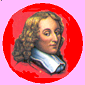### Felix J COLIBRI

+ Home
+ articles_with_sources
+ database
+ web_internet_sockets
+ oop_components
– generics_tutorial
– generics_constraints
– livebindings_spelunking
+ uml_design_patterns
+ debug_and_test
+ graphic
+ controls
+ colibri_utilities
+ colibri_helpers
+ delphi
+ firemonkey
+ compilers